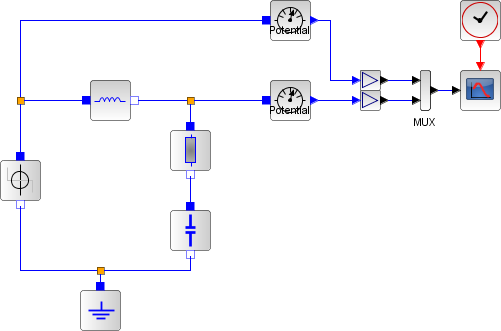# Electrical System modeling

We here consider the following electrical system, with an input voltage Ue and an output voltage Ua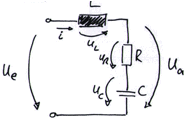## Mathematical modeling

In order to model the system in a mathematical way, we need to use Kirchhoff’s laws:

(1)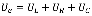(2)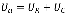In addition, we need to express the mathematical function of each component:

Resistance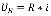Capacity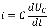Inductor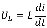Using the 2 mathematical expressions, we come to the following second order differential
equation: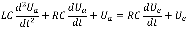## Causal modeling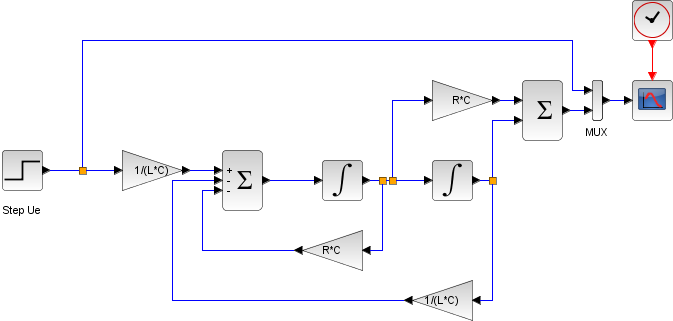## State-space system modeling

The equations for an RLC circuit are the following. They result from
Kirchhoff’s voltage law and Newton’s law.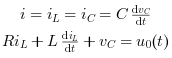The R, L and C are the system’s resistance, inductance
and capacitor.

We define the capacitor voltage Vc and the inductance current iL as the state variables X1 and X2.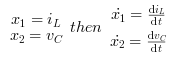thus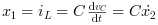Rearranging these equations
we get:These equations can be put into
matrix form as follows,The required
output equation is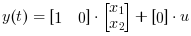The following
diagram shows these equations modeled in Xcos.

To obtain
the output Vc(t) we use CLSS block from Continuous time systems Palette.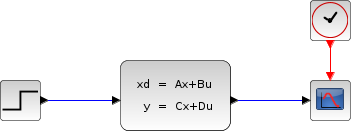https://help.scilab.org/docs/5.5.2/en_US/CLSS.html

## Acausal with Modelica